### Home > CALC > Chapter 5 > Lesson 5.3.1 > Problem5-112

5-112.
1. Differentiate the functions. Homework Help ✎

1.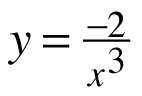2. y = tan(x2 + 3)

3. y = 5(x − 3)8

4.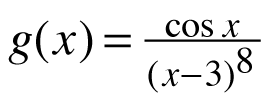5. y = x tan(x2 + 3)

6.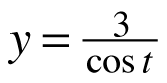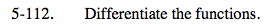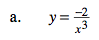Do NOT use the quotient rule (though it will work). This is a Power Rule problem: y = −2x−3. Reserve the Quotient Rule for situations in which there are variables in BOTH the numerator and the denominator.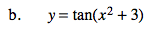Chain Rule.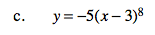This is not technically a Chain Rule problem, though you may use it.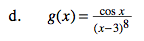This calls for the Quotient Rule.

$g'(x)=\frac{-\text{sin}x(x-3)^{8}-8\text{cos}x(x-3)^7}{(x-3)^{16}}$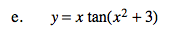Product Rule & Chain Rule combined.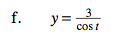Refer to the hint in part (a). And recall that the reciprocal of cosx is secx.